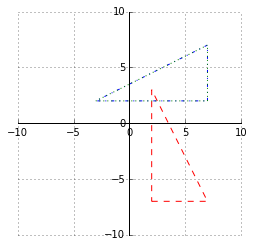# Geometry¶

In :
%matplotlib inline
import math
import numpy as np
import matplotlib.pyplot as plt

def createGrid(breadth): #todo: figure out what this kind o fgrid is called
fig, ax = plt.subplots()
ax.set_aspect('equal')
ax.grid(True)

ax.spines['right'].set_color('none')
ax.spines['top'].set_color('none')

ax.xaxis.set_ticks_position('bottom')
ax.spines['bottom'].set_position(('data',0)) # set position of x spine to x=0

ax.yaxis.set_ticks_position('left')
ax.spines['left'].set_position(('data',0))   # set position of y spine to y=0



## Rotating Shapes¶

The rotation of a positive angle is left <
The rotation of a negative angle is right >

I have to define a shape in code. I think that the lowest way to do this would be a collection of verticies or points.

As an example, this should draw some kind of triangle

[ [2,-7], [7,-7], [2, 3] ]

I can write a function R(numpy.array shape, degreesToRotate theta)

rotation anchor (or center) is assumed to be 0, 0

$$x = cos(theta) * (px-cx) - sin(theta) * (py-cy) + cx$$$$y = sin(theta) * (px-cx) + cos(theta) * (py-cy) + cy$$

func R will only work if I can unpack the numpy.array correctly. The shape [ [2,-7], [7,-7], [2, 3] ] unpacks into 3 args. I need it to unpack into 2 args, where each arg is a set of points in the same dimension.

Fortunately numpy has an easy way to reshape/transform the array.

numpy.array.reshape did NOT produce the correct set.
| 2, -7 |
| 7, -7 |
| 2, 3 |.reshape(2, -1)

returns results sequentially in new matrix bounds (wrong)
| 2, -7, 7 |
|-7, 2, 3 |

numpy.array.T oddly works...
| 2, -7 |
| 7, -7 |
| 2, 3 |.T

returns results based on dimensions (correct)
| 2, 7, 2 |
|-7, -7, 3 |

In :
def R(shape, DegreesToRotate):
cx, cy = 0, 0

Rx = lambda px, py: math.cos(theta) * (px-cx) - math.sin(theta) * (py-cy) + cx
Ry = lambda px, py: math.sin(theta) * (px-cx) + math.cos(theta) * (py-cy) + cy

return np.array([Rx(*shape.T), Ry(*shape.T)]).T

In :
#the original shape
original = np.array([[2,-7], [7,-7], [2, 3]])

rotated = R(original, -270)

answer = np.array([[7, 2], [7, 7], [-3, 2]])

print("computed", rotated)

#boilerplate
createGrid(10)

#just declares the drawable object in memory
triangle = plt.Polygon(original, color='r', fill=0, linestyle='--')
rotatedTri = plt.Polygon(rotated, color='b', fill=0, linestyle='-.')
correctTri = plt.Polygon(answer, color='g', fill=0, linestyle=':')

#actually draw the object(s)

answer [[ 7  2]